# Simulated data

Let’s consider a following problem, the model is defined as

$y = x_1 * x_2 + x_2$

But $$x_1$$ and $$x_2$$ are correlated. How XAI methods work for such model?

# predict function for the model
the_model_predict <- function(m, x) {
x$x1 * x$x2 + x$x2 } # correlated variables N <- 50 set.seed(1) x1 <- runif(N, -5, 5) x2 <- x1 + runif(N)/100 df <- data.frame(x1, x2) # Explainer for the models In fact this model is defined by the predict function the_model_predict. So it does not matter what is in the first argument of the explain function. library("DALEX") explain_the_model <- explain(1, data = df, predict_function = the_model_predict) #> Preparation of a new explainer is initiated #> -> model label : numeric ( [33m default [39m ) #> -> data : 50 rows 2 cols #> -> target variable : not specified! ( [31m WARNING [39m ) #> -> predict function : the_model_predict #> -> predicted values : No value for predict function target column. ( [33m default [39m ) #> -> model_info : package Model of class: numeric package unrecognized , ver. Unknown , task regression ( [33m default [39m ) #> -> model_info : Model info detected regression task but 'y' is a NULL . ( [31m WARNING [39m ) #> -> model_info : By deafult regressions tasks supports only numercical 'y' parameter. #> -> model_info : Consider changing to numerical vector. #> -> model_info : Otherwise I will not be able to calculate residuals or loss function. #> -> predicted values : numerical, min = -0.1726853 , mean = 7.70239 , max = 29.16158 #> -> residual function : difference between y and yhat ( [33m default [39m ) #> [32m A new explainer has been created! [39m # Ceteris paribus Use the ceteris_paribus() function to see Ceteris Paribus profiles. Clearly it’s not an additive model, as the effect of $$x_1$$ depends on $$x_2$$. library("ingredients") library("ggplot2") sample_rows <- data.frame(x1 = -5:5, x2 = -5:5) cp_model <- ceteris_paribus(explain_the_model, sample_rows) plot(cp_model) + show_observations(cp_model) + ggtitle("Ceteris Paribus profiles")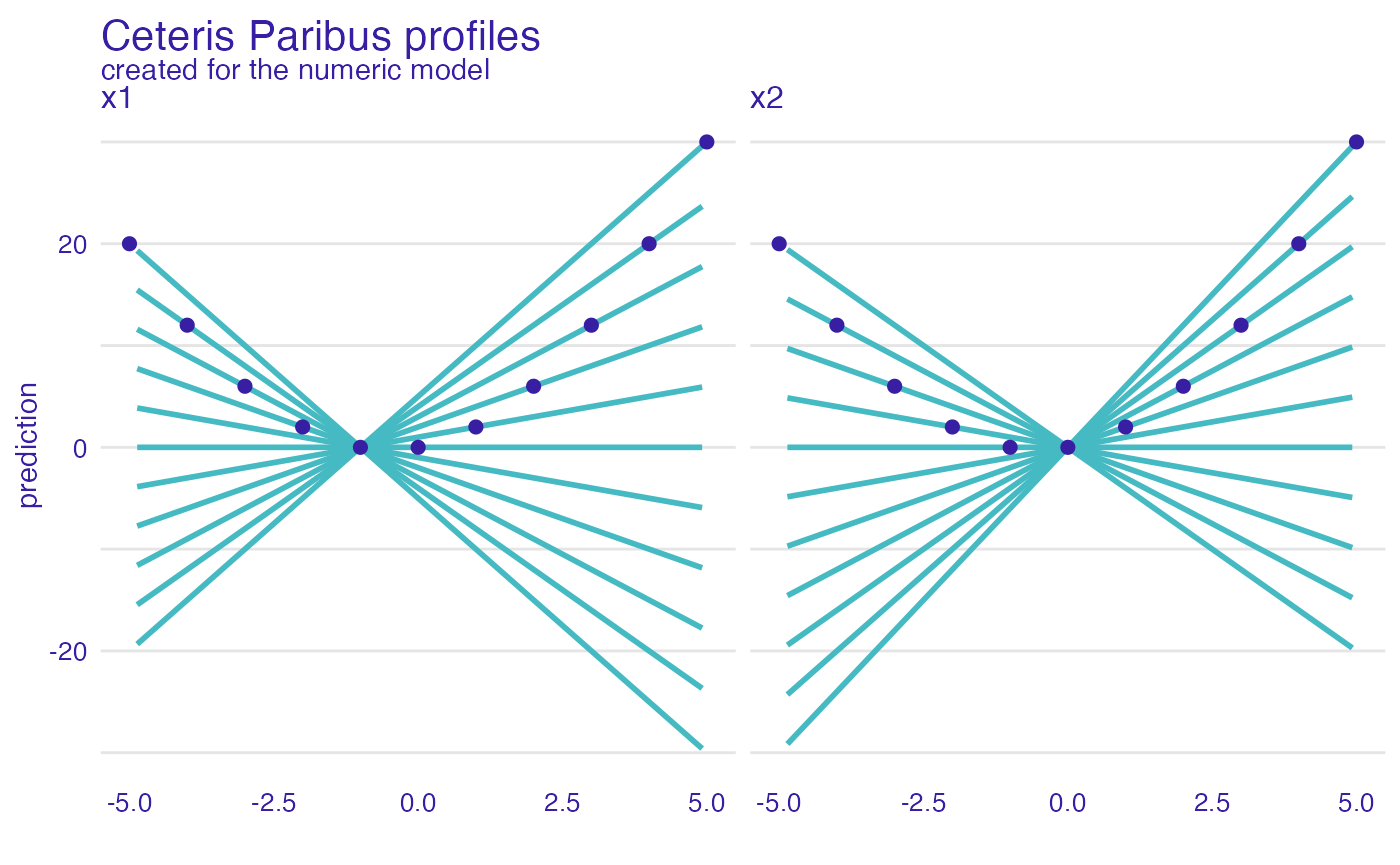# Dependence profiles Lets try Partial Dependence profiles, Conditional Dependence profiles and Accumulated Local profiles. For the last two we can try different smoothing factors pd_model <- partial_dependence(explain_the_model, variables = c("x1", "x2")) pd_model$_label_ = "PDP"

cd_model <- conditional_dependence(explain_the_model, variables = c("x1", "x2"))
cd_model$_label_ = "CDP 0.25" ad_model <- accumulated_dependence(explain_the_model, variables = c("x1", "x2")) ad_model$_label_ = "ALE 0.25"

ggtitle("Feature effects - PDP, CDP, ALE")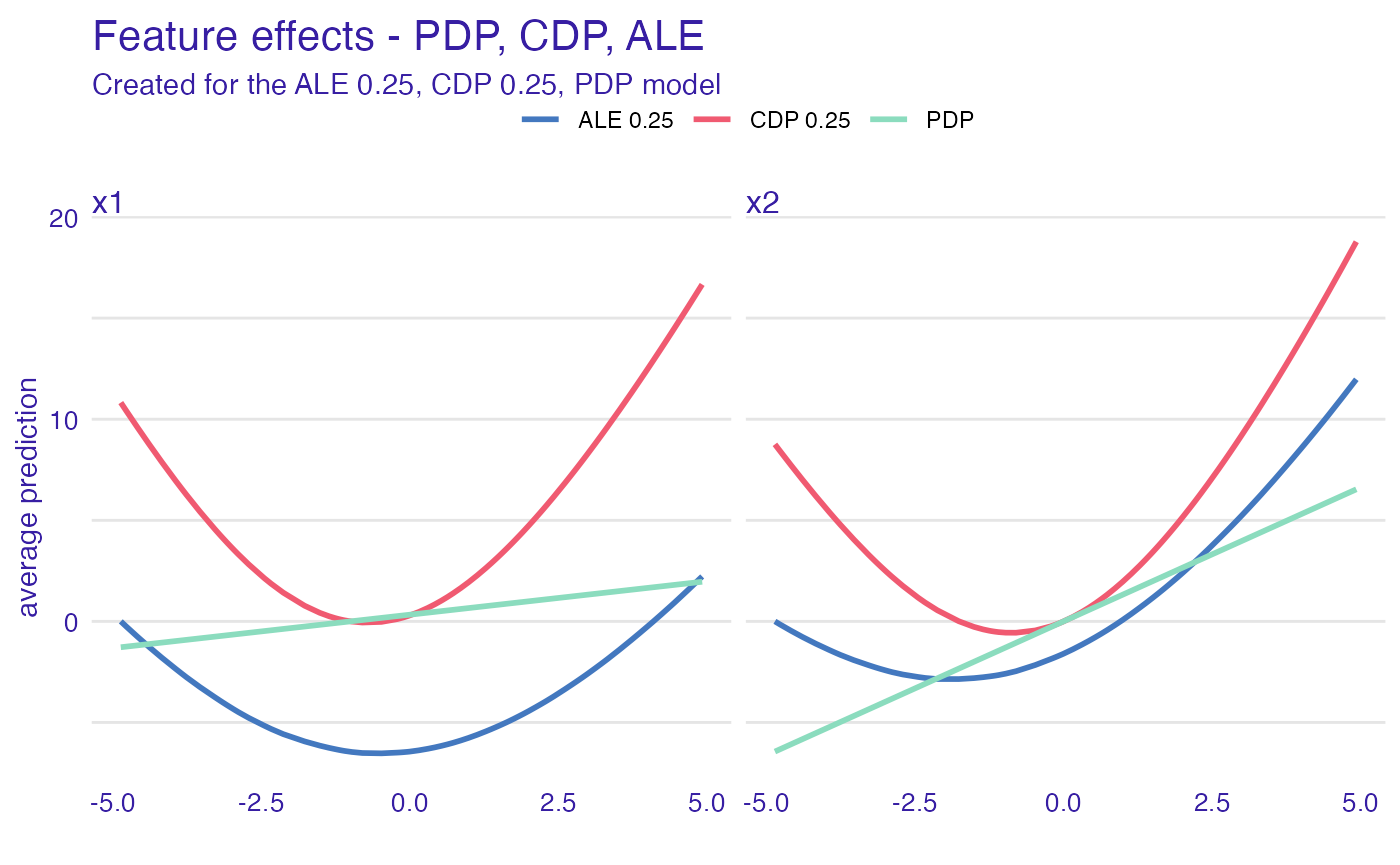cd_model_1 <- conditional_dependence(explain_the_model, variables = c("x1", "x2"), span = 0.1)
cd_model_1$_label_ = "CDP 0.1" cd_model_5 <- conditional_dependence(explain_the_model, variables = c("x1", "x2"), span = 0.5) cd_model_5$_label_ = "CDP 0.5"

ad_model_1 <- accumulated_dependence(explain_the_model, variables = c("x1", "x2"), span = 0.5)
ad_model_1$_label_ = "ALE 0.1" ad_model_5 <- accumulated_dependence(explain_the_model, variables = c("x1", "x2"), span = 0.5) ad_model_5$_label_ = "ALE 0.5"

ggtitle("Feature effects - PDP, CDP, ALE")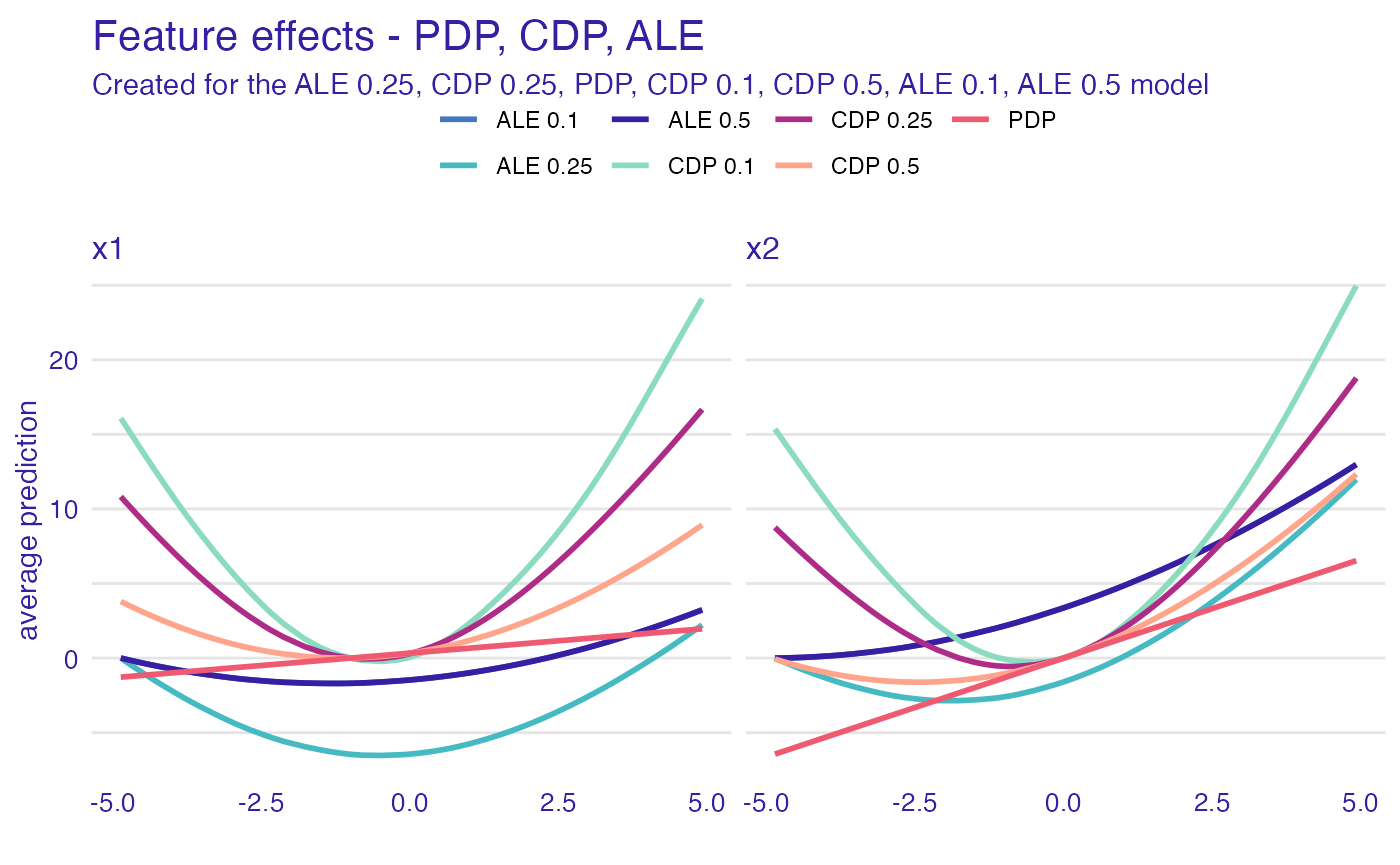# Dependence profiles in groups

And now, let’s see how the grouping factor works

# add grouping variable
df$x3 <- factor(sign(df$x2))
# update the data argument
explain_the_model\$data = df

# PDP in groups
pd_model_groups <- partial_dependence(explain_the_model,
variables = c("x1", "x2"),
groups = "x3")
plot(pd_model_groups) +
ggtitle("Partial Dependence")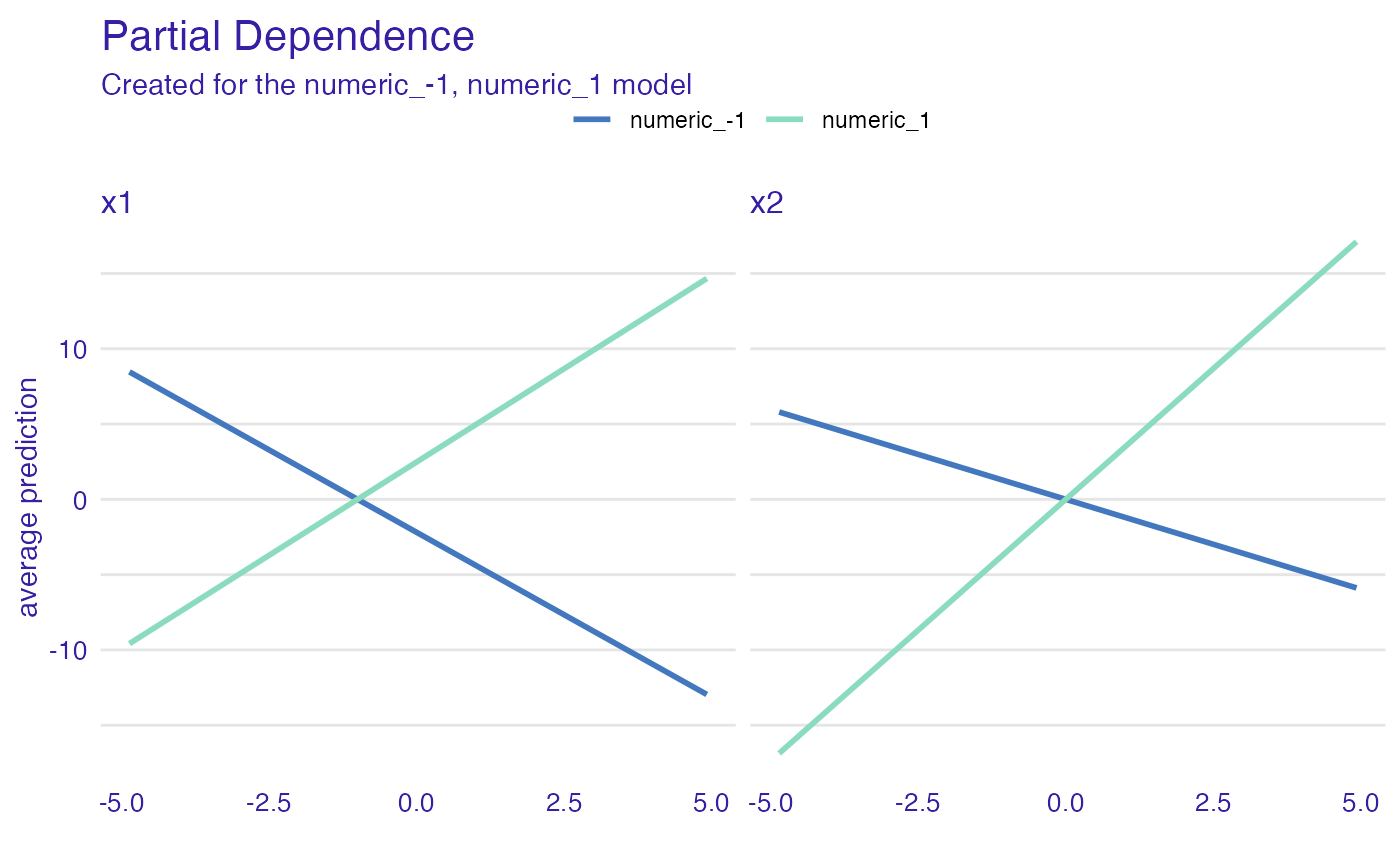# ALE in groups
variables = c("x1", "x2"),
groups = "x3")
ggtitle("Accumulated Local")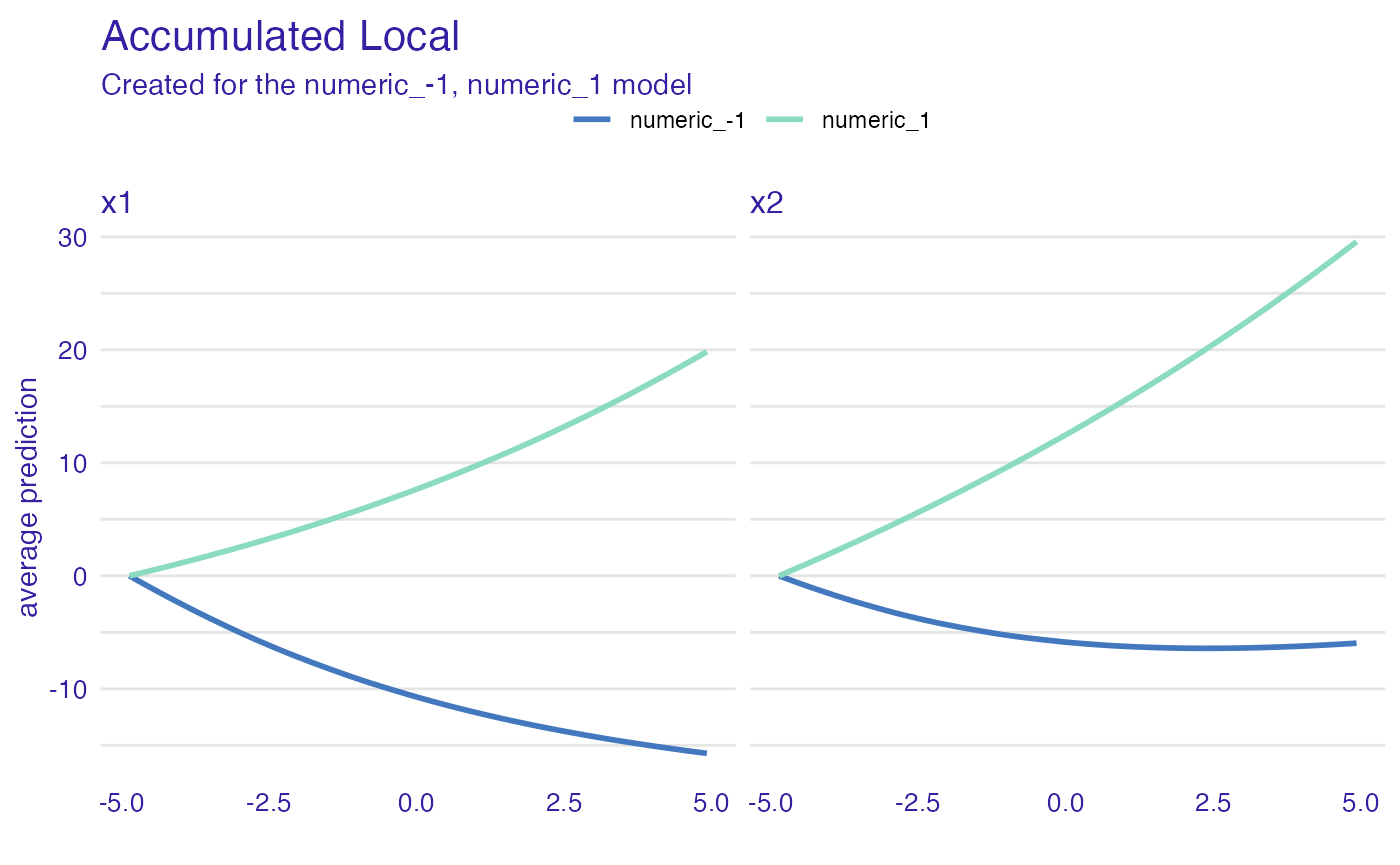# CDP in groups
cd_model_groups <- conditional_dependence(explain_the_model,
variables = c("x1", "x2"),
groups = "x3")
plot(cd_model_groups) +
ggtitle("Conditional Dependence")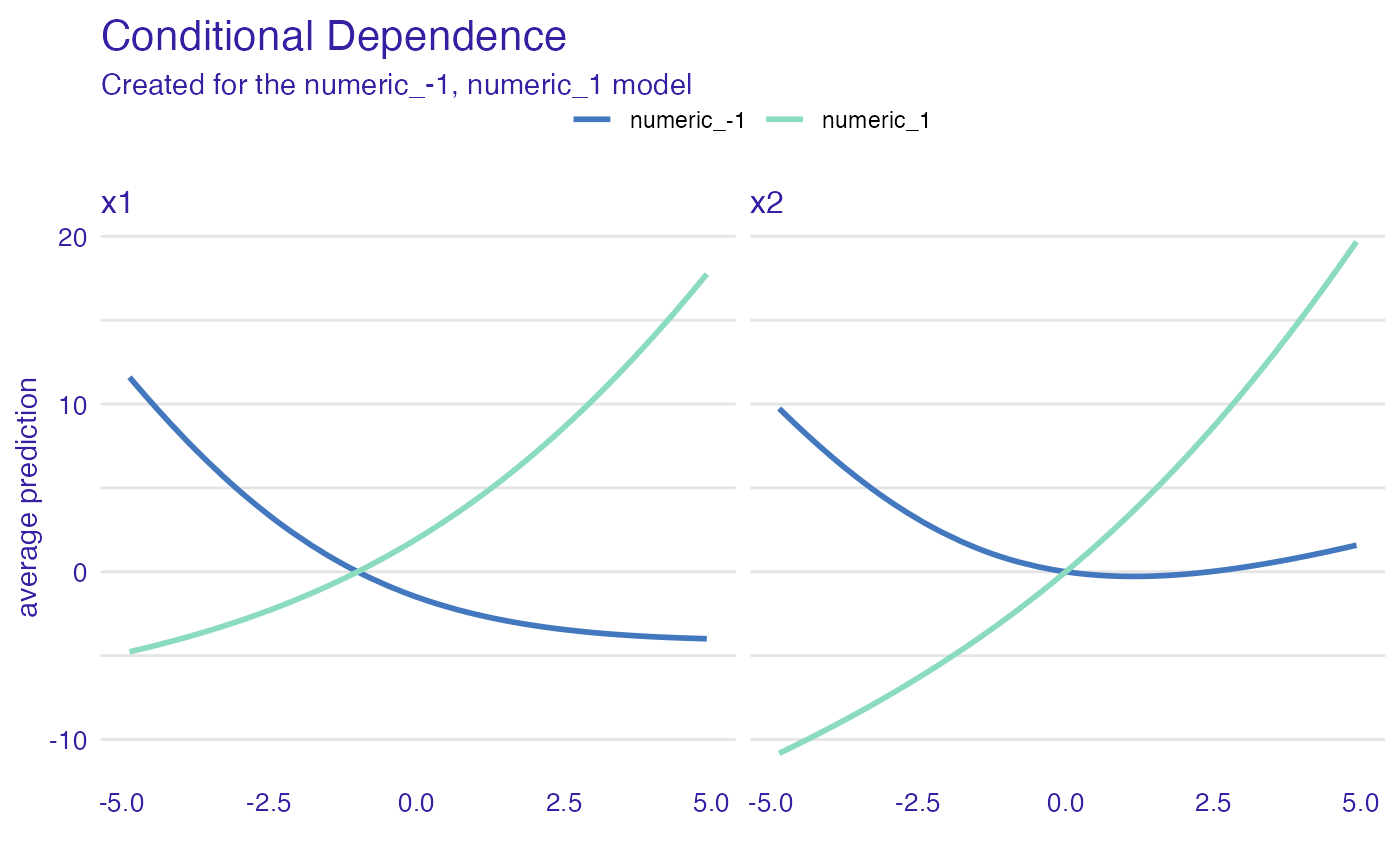# Session info

sessionInfo()
#> R version 4.1.1 (2021-08-10)
#> Platform: x86_64-apple-darwin17.0 (64-bit)
#> Running under: macOS Catalina 10.15.7
#>
#> Matrix products: default
#> BLAS:   /Library/Frameworks/R.framework/Versions/4.1/Resources/lib/libRblas.0.dylib
#> LAPACK: /Library/Frameworks/R.framework/Versions/4.1/Resources/lib/libRlapack.dylib
#>
#> locale:
#>  en_US.UTF-8/en_US.UTF-8/en_US.UTF-8/C/en_US.UTF-8/en_US.UTF-8
#>
#> attached base packages:
#>  stats     graphics  grDevices utils     datasets  methods   base
#>
#> other attached packages:
#>  ggplot2_3.3.5     ingredients_2.2.1 DALEX_2.3.0
#>
#> loaded via a namespace (and not attached):
#>   highr_0.9         compiler_4.1.1    pillar_1.6.3      jquerylib_0.1.4
#>   tools_4.1.1       digest_0.6.28     evaluate_0.14     memoise_2.0.0
#>   lifecycle_1.0.1   tibble_3.1.5      gtable_0.3.0      pkgconfig_2.0.3
#>  rlang_0.4.11      yaml_2.2.1        pkgdown_1.6.1     xfun_0.26
#>  fastmap_1.1.0     withr_2.4.2       stringr_1.4.0     knitr_1.36
#>  desc_1.4.0        fs_1.5.0          vctrs_0.3.8       systemfonts_1.0.2
#>  rprojroot_2.0.2   grid_4.1.1        glue_1.4.2        R6_2.5.1
#>  textshaping_0.3.5 fansi_0.5.0       rmarkdown_2.11    farver_2.1.0
#>  magrittr_2.0.1    scales_1.1.1      htmltools_0.5.2   ellipsis_0.3.2
#>  colorspace_2.0-2  labeling_0.4.2    ragg_1.1.3        utf8_1.2.2
#>  stringi_1.7.5     munsell_0.5.0     cachem_1.0.6      crayon_1.4.1